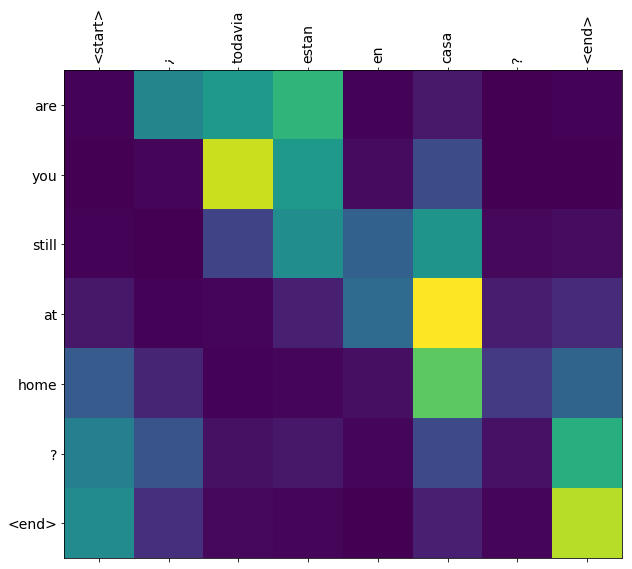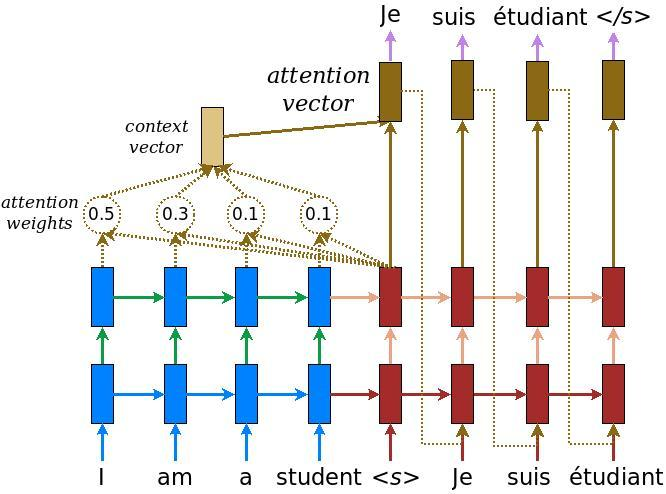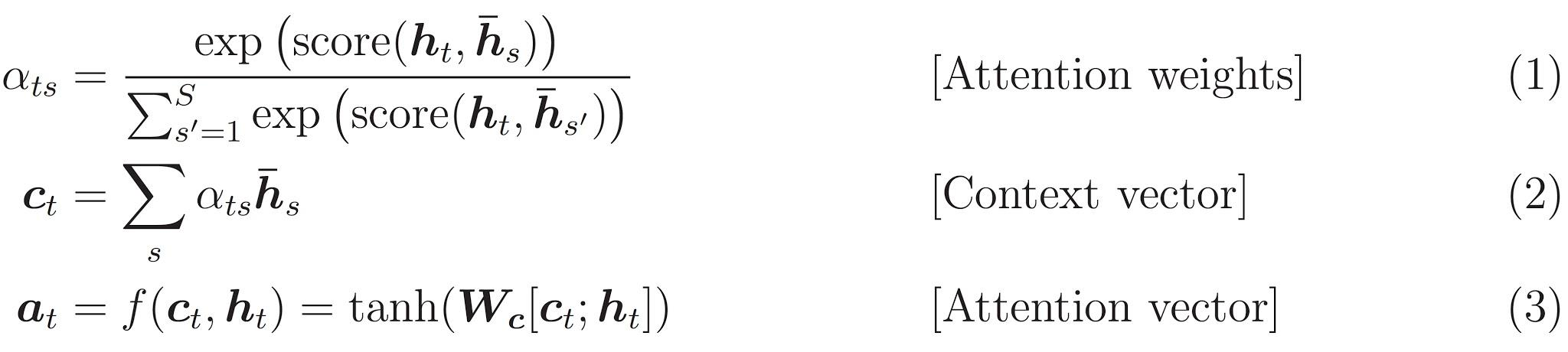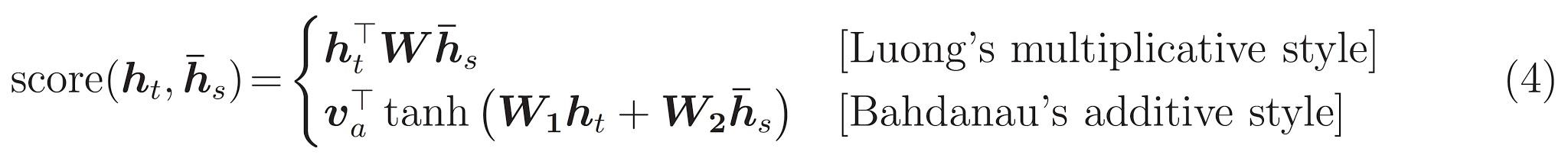# Neural machine translation with attention

This notebook trains a sequence to sequence (seq2seq) model for Spanish to English translation. This is an advanced example that assumes some knowledge of sequence to sequence models.

After training the model in this notebook, you will be able to input a Spanish sentence, such as "¿todavia estan en casa?", and return the English translation: "are you still at home?"

The translation quality is reasonable for a toy example, but the generated attention plot is perhaps more interesting. This shows which parts of the input sentence has the model's attention while translating:``````from __future__ import absolute_import, division, print_function, unicode_literals

import tensorflow as tf

import matplotlib.pyplot as plt
import matplotlib.ticker as ticker
from sklearn.model_selection import train_test_split

import unicodedata
import re
import numpy as np
import os
import io
import time
``````

We'll use a language dataset provided by http://www.manythings.org/anki/. This dataset contains language translation pairs in the format:

``````May I borrow this book? ¿Puedo tomar prestado este libro?
``````

There are a variety of languages available, but we'll use the English-Spanish dataset. For convenience, we've hosted a copy of this dataset on Google Cloud, but you can also download your own copy. After downloading the dataset, here are the steps we'll take to prepare the data:

1. Add a start and end token to each sentence.
2. Clean the sentences by removing special characters.
3. Create a word index and reverse word index (dictionaries mapping from word → id and id → word).
4. Pad each sentence to a maximum length.
``````# Download the file
path_to_zip = tf.keras.utils.get_file(
extract=True)

path_to_file = os.path.dirname(path_to_zip)+"/spa-eng/spa.txt"
``````
```Downloading data from http://storage.googleapis.com/download.tensorflow.org/data/spa-eng.zip
2646016/2638744 [==============================] - 0s 0us/step
```
``````# Converts the unicode file to ascii
def unicode_to_ascii(s):
return ''.join(c for c in unicodedata.normalize('NFD', s)
if unicodedata.category(c) != 'Mn')

def preprocess_sentence(w):
w = unicode_to_ascii(w.lower().strip())

# creating a space between a word and the punctuation following it
# eg: "he is a boy." => "he is a boy ."
w = re.sub(r"([?.!,¿])", r" \1 ", w)
w = re.sub(r'[" "]+', " ", w)

# replacing everything with space except (a-z, A-Z, ".", "?", "!", ",")
w = re.sub(r"[^a-zA-Z?.!,¿]+", " ", w)

w = w.rstrip().strip()

# adding a start and an end token to the sentence
# so that the model know when to start and stop predicting.
w = '<start> ' + w + ' <end>'
return w
``````
``````en_sentence = u"May I borrow this book?"
sp_sentence = u"¿Puedo tomar prestado este libro?"
print(preprocess_sentence(en_sentence))
print(preprocess_sentence(sp_sentence).encode('utf-8'))
``````
```<start> may i borrow this book ? <end>
b'<start> \xc2\xbf puedo tomar prestado este libro ? <end>'
```
``````# 1. Remove the accents
# 2. Clean the sentences
# 3. Return word pairs in the format: [ENGLISH, SPANISH]
def create_dataset(path, num_examples):

word_pairs = [[preprocess_sentence(w) for w in l.split('\t')]  for l in lines[:num_examples]]

return zip(*word_pairs)
``````
``````en, sp = create_dataset(path_to_file, None)
print(en[-1])
print(sp[-1])
``````
```<start> if you want to sound like a native speaker , you must be willing to practice saying the same sentence over and over in the same way that banjo players practice the same phrase over and over until they can play it correctly and at the desired tempo . <end>
<start> si quieres sonar como un hablante nativo , debes estar dispuesto a practicar diciendo la misma frase una y otra vez de la misma manera en que un musico de banjo practica el mismo fraseo una y otra vez hasta que lo puedan tocar correctamente y en el tiempo esperado . <end>
```
``````def max_length(tensor):
return max(len(t) for t in tensor)
``````
``````def tokenize(lang):
lang_tokenizer = tf.keras.preprocessing.text.Tokenizer(
filters='')
lang_tokenizer.fit_on_texts(lang)

tensor = lang_tokenizer.texts_to_sequences(lang)

return tensor, lang_tokenizer
``````
``````def load_dataset(path, num_examples=None):
# creating cleaned input, output pairs
targ_lang, inp_lang = create_dataset(path, num_examples)

input_tensor, inp_lang_tokenizer = tokenize(inp_lang)
target_tensor, targ_lang_tokenizer = tokenize(targ_lang)

return input_tensor, target_tensor, inp_lang_tokenizer, targ_lang_tokenizer
``````

### Limit the size of the dataset to experiment faster (optional)

Training on the complete dataset of >100,000 sentences will take a long time. To train faster, we can limit the size of the dataset to 30,000 sentences (of course, translation quality degrades with less data):

``````# Try experimenting with the size of that dataset
num_examples = 30000
input_tensor, target_tensor, inp_lang, targ_lang = load_dataset(path_to_file, num_examples)

# Calculate max_length of the target tensors
max_length_targ, max_length_inp = max_length(target_tensor), max_length(input_tensor)
``````
``````# Creating training and validation sets using an 80-20 split
input_tensor_train, input_tensor_val, target_tensor_train, target_tensor_val = train_test_split(input_tensor, target_tensor, test_size=0.2)

# Show length
print(len(input_tensor_train), len(target_tensor_train), len(input_tensor_val), len(target_tensor_val))
``````
```24000 24000 6000 6000
```
``````def convert(lang, tensor):
for t in tensor:
if t!=0:
print ("%d ----> %s" % (t, lang.index_word[t]))
``````
``````print ("Input Language; index to word mapping")
convert(inp_lang, input_tensor_train)
print ()
print ("Target Language; index to word mapping")
convert(targ_lang, target_tensor_train)
``````
```Input Language; index to word mapping
1 ----> <start>
265 ----> compre
15 ----> un
6648 ----> nopal
3 ----> .
2 ----> <end>

Target Language; index to word mapping
1 ----> <start>
4 ----> i
222 ----> bought
9 ----> a
2787 ----> cactus
3 ----> .
2 ----> <end>
```

### Create a tf.data dataset

``````BUFFER_SIZE = len(input_tensor_train)
BATCH_SIZE = 64
steps_per_epoch = len(input_tensor_train)//BATCH_SIZE
embedding_dim = 256
units = 1024
vocab_inp_size = len(inp_lang.word_index)+1
vocab_tar_size = len(targ_lang.word_index)+1

dataset = tf.data.Dataset.from_tensor_slices((input_tensor_train, target_tensor_train)).shuffle(BUFFER_SIZE)
dataset = dataset.batch(BATCH_SIZE, drop_remainder=True)
``````
``````example_input_batch, example_target_batch = next(iter(dataset))
example_input_batch.shape, example_target_batch.shape
``````
`(TensorShape([64, 16]), TensorShape([64, 11]))`

## Write the encoder and decoder model

Implement an encoder-decoder model with attention which you can read about in the TensorFlow Neural Machine Translation (seq2seq) tutorial. This example uses a more recent set of APIs. This notebook implements the attention equations from the seq2seq tutorial. The following diagram shows that each input words is assigned a weight by the attention mechanism which is then used by the decoder to predict the next word in the sentence. The below picture and formulas are an example of attention mechanism from Luong's paper.The input is put through an encoder model which gives us the encoder output of shape (batch_size, max_length, hidden_size) and the encoder hidden state of shape (batch_size, hidden_size).

Here are the equations that are implemented:This tutorial uses Bahdanau attention for the encoder. Let's decide on notation before writing the simplified form:

• FC = Fully connected (dense) layer
• EO = Encoder output
• H = hidden state
• X = input to the decoder

And the pseudo-code:

• `score = FC(tanh(FC(EO) + FC(H)))`
• `attention weights = softmax(score, axis = 1)`. Softmax by default is applied on the last axis but here we want to apply it on the 1st axis, since the shape of score is (batch_size, max_length, hidden_size). `Max_length` is the length of our input. Since we are trying to assign a weight to each input, softmax should be applied on that axis.
• `context vector = sum(attention weights * EO, axis = 1)`. Same reason as above for choosing axis as 1.
• `embedding output` = The input to the decoder X is passed through an embedding layer.
• `merged vector = concat(embedding output, context vector)`
• This merged vector is then given to the GRU

The shapes of all the vectors at each step have been specified in the comments in the code:

``````class Encoder(tf.keras.Model):
def __init__(self, vocab_size, embedding_dim, enc_units, batch_sz):
super(Encoder, self).__init__()
self.batch_sz = batch_sz
self.enc_units = enc_units
self.embedding = tf.keras.layers.Embedding(vocab_size, embedding_dim)
self.gru = tf.keras.layers.GRU(self.enc_units,
return_sequences=True,
return_state=True,
recurrent_initializer='glorot_uniform')

def call(self, x, hidden):
x = self.embedding(x)
output, state = self.gru(x, initial_state = hidden)
return output, state

def initialize_hidden_state(self):
return tf.zeros((self.batch_sz, self.enc_units))
``````
``````encoder = Encoder(vocab_inp_size, embedding_dim, units, BATCH_SIZE)

# sample input
sample_hidden = encoder.initialize_hidden_state()
sample_output, sample_hidden = encoder(example_input_batch, sample_hidden)
print ('Encoder output shape: (batch size, sequence length, units) {}'.format(sample_output.shape))
print ('Encoder Hidden state shape: (batch size, units) {}'.format(sample_hidden.shape))
``````
```Encoder output shape: (batch size, sequence length, units) (64, 16, 1024)
Encoder Hidden state shape: (batch size, units) (64, 1024)
```
``````class BahdanauAttention(tf.keras.layers.Layer):
def __init__(self, units):
super(BahdanauAttention, self).__init__()
self.W1 = tf.keras.layers.Dense(units)
self.W2 = tf.keras.layers.Dense(units)
self.V = tf.keras.layers.Dense(1)

def call(self, query, values):
# hidden shape == (batch_size, hidden size)
# hidden_with_time_axis shape == (batch_size, 1, hidden size)
# we are doing this to perform addition to calculate the score
hidden_with_time_axis = tf.expand_dims(query, 1)

# score shape == (batch_size, max_length, 1)
# we get 1 at the last axis because we are applying score to self.V
# the shape of the tensor before applying self.V is (batch_size, max_length, units)
score = self.V(tf.nn.tanh(
self.W1(values) + self.W2(hidden_with_time_axis)))

# attention_weights shape == (batch_size, max_length, 1)
attention_weights = tf.nn.softmax(score, axis=1)

# context_vector shape after sum == (batch_size, hidden_size)
context_vector = attention_weights * values
context_vector = tf.reduce_sum(context_vector, axis=1)

return context_vector, attention_weights
``````
``````attention_layer = BahdanauAttention(10)
attention_result, attention_weights = attention_layer(sample_hidden, sample_output)

print("Attention result shape: (batch size, units) {}".format(attention_result.shape))
print("Attention weights shape: (batch_size, sequence_length, 1) {}".format(attention_weights.shape))
``````
```Attention result shape: (batch size, units) (64, 1024)
Attention weights shape: (batch_size, sequence_length, 1) (64, 16, 1)
```
``````class Decoder(tf.keras.Model):
def __init__(self, vocab_size, embedding_dim, dec_units, batch_sz):
super(Decoder, self).__init__()
self.batch_sz = batch_sz
self.dec_units = dec_units
self.embedding = tf.keras.layers.Embedding(vocab_size, embedding_dim)
self.gru = tf.keras.layers.GRU(self.dec_units,
return_sequences=True,
return_state=True,
recurrent_initializer='glorot_uniform')
self.fc = tf.keras.layers.Dense(vocab_size)

# used for attention
self.attention = BahdanauAttention(self.dec_units)

def call(self, x, hidden, enc_output):
# enc_output shape == (batch_size, max_length, hidden_size)
context_vector, attention_weights = self.attention(hidden, enc_output)

# x shape after passing through embedding == (batch_size, 1, embedding_dim)
x = self.embedding(x)

# x shape after concatenation == (batch_size, 1, embedding_dim + hidden_size)
x = tf.concat([tf.expand_dims(context_vector, 1), x], axis=-1)

# passing the concatenated vector to the GRU
output, state = self.gru(x)

# output shape == (batch_size * 1, hidden_size)
output = tf.reshape(output, (-1, output.shape))

# output shape == (batch_size, vocab)
x = self.fc(output)

return x, state, attention_weights
``````
``````decoder = Decoder(vocab_tar_size, embedding_dim, units, BATCH_SIZE)

sample_decoder_output, _, _ = decoder(tf.random.uniform((BATCH_SIZE, 1)),
sample_hidden, sample_output)

print ('Decoder output shape: (batch_size, vocab size) {}'.format(sample_decoder_output.shape))
``````
```Decoder output shape: (batch_size, vocab size) (64, 4935)
```

## Define the optimizer and the loss function

``````optimizer = tf.keras.optimizers.Adam()
loss_object = tf.keras.losses.SparseCategoricalCrossentropy(
from_logits=True, reduction='none')

def loss_function(real, pred):
loss_ = loss_object(real, pred)

return tf.reduce_mean(loss_)
``````

## Checkpoints (Object-based saving)

``````checkpoint_dir = './training_checkpoints'
checkpoint_prefix = os.path.join(checkpoint_dir, "ckpt")
checkpoint = tf.train.Checkpoint(optimizer=optimizer,
encoder=encoder,
decoder=decoder)
``````

## Training

1. Pass the input through the encoder which return encoder output and the encoder hidden state.
2. The encoder output, encoder hidden state and the decoder input (which is the start token) is passed to the decoder.
3. The decoder returns the predictions and the decoder hidden state.
4. The decoder hidden state is then passed back into the model and the predictions are used to calculate the loss.
5. Use teacher forcing to decide the next input to the decoder.
6. Teacher forcing is the technique where the target word is passed as the next input to the decoder.
7. The final step is to calculate the gradients and apply it to the optimizer and backpropagate.
``````@tf.function
def train_step(inp, targ, enc_hidden):
loss = 0

enc_output, enc_hidden = encoder(inp, enc_hidden)

dec_hidden = enc_hidden

dec_input = tf.expand_dims([targ_lang.word_index['<start>']] * BATCH_SIZE, 1)

# Teacher forcing - feeding the target as the next input
for t in range(1, targ.shape):
# passing enc_output to the decoder
predictions, dec_hidden, _ = decoder(dec_input, dec_hidden, enc_output)

loss += loss_function(targ[:, t], predictions)

# using teacher forcing
dec_input = tf.expand_dims(targ[:, t], 1)

batch_loss = (loss / int(targ.shape))

variables = encoder.trainable_variables + decoder.trainable_variables

return batch_loss
``````
``````EPOCHS = 10

for epoch in range(EPOCHS):
start = time.time()

enc_hidden = encoder.initialize_hidden_state()
total_loss = 0

for (batch, (inp, targ)) in enumerate(dataset.take(steps_per_epoch)):
batch_loss = train_step(inp, targ, enc_hidden)
total_loss += batch_loss

if batch % 100 == 0:
print('Epoch {} Batch {} Loss {:.4f}'.format(epoch + 1,
batch,
batch_loss.numpy()))
# saving (checkpoint) the model every 2 epochs
if (epoch + 1) % 2 == 0:
checkpoint.save(file_prefix = checkpoint_prefix)

print('Epoch {} Loss {:.4f}'.format(epoch + 1,
total_loss / steps_per_epoch))
print('Time taken for 1 epoch {} sec\n'.format(time.time() - start))
``````
```Epoch 1 Batch 0 Loss 4.7473
Epoch 1 Batch 100 Loss 2.1733
Epoch 1 Batch 200 Loss 1.8787
Epoch 1 Batch 300 Loss 1.7222
Epoch 1 Loss 2.0324
Time taken for 1 epoch 33.9479820728302 sec

Epoch 2 Batch 0 Loss 1.5579
Epoch 2 Batch 100 Loss 1.4370
Epoch 2 Batch 200 Loss 1.4042
Epoch 2 Batch 300 Loss 1.2848
Epoch 2 Loss 1.3962
Time taken for 1 epoch 17.57913875579834 sec

Epoch 3 Batch 0 Loss 1.0146
Epoch 3 Batch 100 Loss 0.9916
Epoch 3 Batch 200 Loss 1.0946
Epoch 3 Batch 300 Loss 0.9436
Epoch 3 Loss 0.9849
Time taken for 1 epoch 17.110281229019165 sec

Epoch 4 Batch 0 Loss 0.6478
Epoch 4 Batch 100 Loss 0.6424
Epoch 4 Batch 200 Loss 0.7041
Epoch 4 Batch 300 Loss 0.6307
Epoch 4 Loss 0.6634
Time taken for 1 epoch 17.549187421798706 sec

Epoch 5 Batch 0 Loss 0.4491
Epoch 5 Batch 100 Loss 0.3657
Epoch 5 Batch 200 Loss 0.4612
Epoch 5 Batch 300 Loss 0.4688
Epoch 5 Loss 0.4508
Time taken for 1 epoch 17.02736186981201 sec

Epoch 6 Batch 0 Loss 0.3293
Epoch 6 Batch 100 Loss 0.3074
Epoch 6 Batch 200 Loss 0.3077
Epoch 6 Batch 300 Loss 0.2849
Epoch 6 Loss 0.3147
Time taken for 1 epoch 17.503292560577393 sec

Epoch 7 Batch 0 Loss 0.1896
Epoch 7 Batch 100 Loss 0.2028
Epoch 7 Batch 200 Loss 0.2070
Epoch 7 Batch 300 Loss 0.1809
Epoch 7 Loss 0.2236
Time taken for 1 epoch 17.121249437332153 sec

Epoch 8 Batch 0 Loss 0.1307
Epoch 8 Batch 100 Loss 0.2151
Epoch 8 Batch 200 Loss 0.1595
Epoch 8 Batch 300 Loss 0.1825
Epoch 8 Loss 0.1683
Time taken for 1 epoch 17.56266689300537 sec

Epoch 9 Batch 0 Loss 0.1091
Epoch 9 Batch 100 Loss 0.1238
Epoch 9 Batch 200 Loss 0.1262
Epoch 9 Batch 300 Loss 0.1245
Epoch 9 Loss 0.1290
Time taken for 1 epoch 17.148908615112305 sec

Epoch 10 Batch 0 Loss 0.0939
Epoch 10 Batch 100 Loss 0.1082
Epoch 10 Batch 200 Loss 0.1149
Epoch 10 Batch 300 Loss 0.0957
Epoch 10 Loss 0.1038
Time taken for 1 epoch 17.501878261566162 sec

```

## Translate

• The evaluate function is similar to the training loop, except we don't use teacher forcing here. The input to the decoder at each time step is its previous predictions along with the hidden state and the encoder output.
• Stop predicting when the model predicts the end token.
• And store the attention weights for every time step.
``````def evaluate(sentence):
attention_plot = np.zeros((max_length_targ, max_length_inp))

sentence = preprocess_sentence(sentence)

inputs = [inp_lang.word_index[i] for i in sentence.split(' ')]
maxlen=max_length_inp,
inputs = tf.convert_to_tensor(inputs)

result = ''

hidden = [tf.zeros((1, units))]
enc_out, enc_hidden = encoder(inputs, hidden)

dec_hidden = enc_hidden
dec_input = tf.expand_dims([targ_lang.word_index['<start>']], 0)

for t in range(max_length_targ):
predictions, dec_hidden, attention_weights = decoder(dec_input,
dec_hidden,
enc_out)

# storing the attention weights to plot later on
attention_weights = tf.reshape(attention_weights, (-1, ))
attention_plot[t] = attention_weights.numpy()

predicted_id = tf.argmax(predictions).numpy()

result += targ_lang.index_word[predicted_id] + ' '

if targ_lang.index_word[predicted_id] == '<end>':
return result, sentence, attention_plot

# the predicted ID is fed back into the model
dec_input = tf.expand_dims([predicted_id], 0)

return result, sentence, attention_plot
``````
``````# function for plotting the attention weights
def plot_attention(attention, sentence, predicted_sentence):
fig = plt.figure(figsize=(10,10))
ax.matshow(attention, cmap='viridis')

fontdict = {'fontsize': 14}

ax.set_xticklabels([''] + sentence, fontdict=fontdict, rotation=90)
ax.set_yticklabels([''] + predicted_sentence, fontdict=fontdict)

ax.xaxis.set_major_locator(ticker.MultipleLocator(1))
ax.yaxis.set_major_locator(ticker.MultipleLocator(1))

plt.show()
``````
``````def translate(sentence):
result, sentence, attention_plot = evaluate(sentence)

print('Input: %s' % (sentence))
print('Predicted translation: {}'.format(result))

attention_plot = attention_plot[:len(result.split(' ')), :len(sentence.split(' '))]
plot_attention(attention_plot, sentence.split(' '), result.split(' '))
``````

## Restore the latest checkpoint and test

``````# restoring the latest checkpoint in checkpoint_dir
checkpoint.restore(tf.train.latest_checkpoint(checkpoint_dir))
``````
`<tensorflow.python.training.tracking.util.CheckpointLoadStatus at 0x7f494a9dcbe0>`
``````translate(u'hace mucho frio aqui.')
``````
```Input: <start> hace mucho frio aqui . <end>
Predicted translation: it s very cold here . <end>
`````````translate(u'esta es mi vida.')
``````
```Input: <start> esta es mi vida . <end>
Predicted translation: this is my life . <end>
`````````translate(u'¿todavia estan en casa?')
``````
```Input: <start> ¿ todavia estan en casa ? <end>
Predicted translation: are you still at home ? <end>
`````````# wrong translation
translate(u'trata de averiguarlo.')
``````
```Input: <start> trata de averiguarlo . <end>
Predicted translation: try to figure it out . <end>
```## Next steps

• Download a different dataset to experiment with translations, for example, English to German, or English to French.
• Experiment with training on a larger dataset, or using more epochs